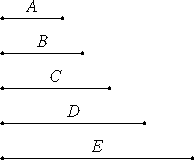# Proposition 17

If there are as many numbers as we please in continued proportion, and the extremes of them are relatively prime, then the last is not to any other number as the first is to the second.

Let there be as many numbers as we please, A, B, C, and D, in continued proportion, and let the extremes of them, A and D, be relatively prime.

I say that D is not to any other number as A is to B.VII.13

If possible A is to B, so let D be to E, therefore, alternately A is to D as B is to E.

But A and D are prime, primes are also least, and the least numbers measure those which have the same ratio the same number of times, the antecedent the antecedent and the consequent the consequent. Therefore A measures B. And A is to B as B is to C. Therefore B also measures C, so that A also measures C.

And since B is to C as C is to D, and B measures C, therefore C also measures D. But A measures C, so that A also measures D. But it also measures itself, therefore A measures A and D which are relatively prime, which is impossible.

Therefore D is not to any other number as A is to B.

Therefore, if there are as many numbers as we please in continued proportion, and the extremes of them are relatively prime, then the last is not to any other number as the first is to the second.

Q.E.D.

## Guide

This proposition generalizes the previous proposition from a ratio of two terms to a continued proportion of arbitrarily many. It says that a continued proportion in lowest terms cannot be extended.

#### Outline of the proof

Consider a continued proportion in lowest terms with the first term a relatively prime to the last term d, and having the ratio a : b. Suppose it can be extended to e so that a : b = d : e. Alternately, a : d = b : e. Since the first ratio a : d is in lowest terms, therefore a divides b. Then each term of the continued proportion divides the next, hence a divides d. But that’s impossible since a and d are relatively prime. Therefore, the continued proportion cannot be extended.